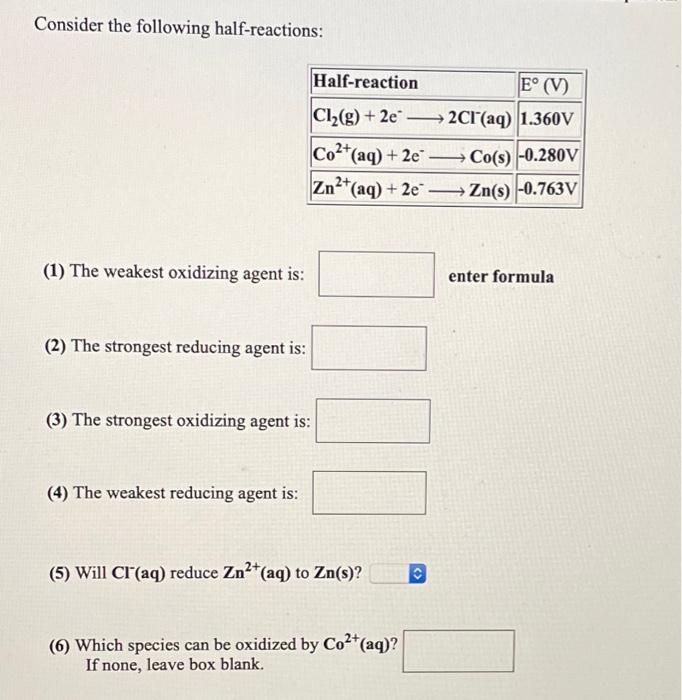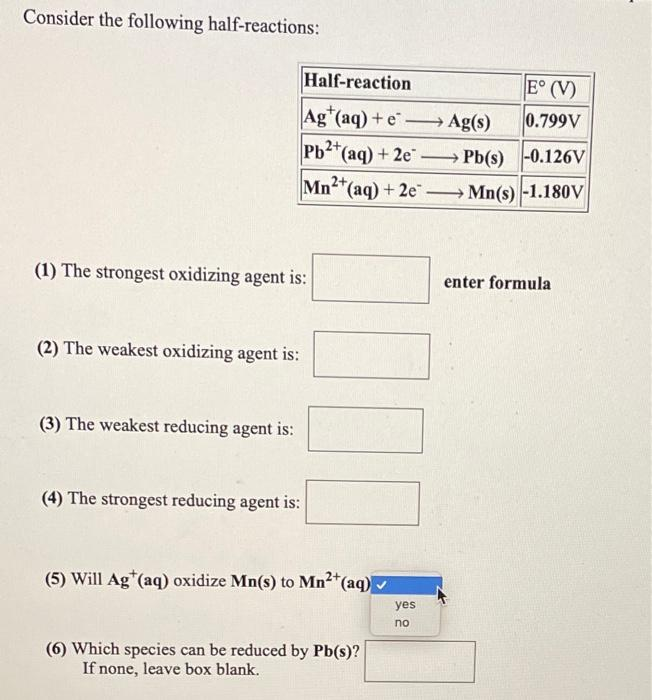# Question Solved1 AnswerConsider the following half-reactions: Half-reaction E° (V) Cl2(g) + 2e 2Cl(aq) 1.360V Co2+(aq) + 2e Co(s) -0.280V Zn2+(aq) + 2e = Zn(s) -0.763V → (1) The weakest oxidizing agent is: enter formula (2) The strongest reducing agent is: (3) The strongest oxidizing agent is: (4) The weakest reducing agent is: (5) Will Cl(aq) reduce Zn2+ (aq) to Zn(s)? Co2+(aq)? () Which species can be oxidized by If none, leave box blank. Consider the following half-reactions: Half-reaction E° (V) Ag+ (aq) + 2Ag(s) 0.799V Pb2+(aq) + 2e → Pb(s) -0.126V Mn? 12+(aq) + 2e —— Mn(s) -1.180V (1) The strongest oxidizing agent is: enter formula (2) The weakest oxidizing agent is: (3) The weakest reducing agent is: (4) The strongest reducing agent is: (5) Will Agt(aq) oxidize Mn(s) to Mn2+(aq) yes no (6) Which species can be reduced by Pb(s)? If none, leave box blank.Transcribed Image Text: Consider the following half-reactions: Half-reaction E° (V) Cl2(g) + 2e 2Cl(aq) 1.360V Co2+(aq) + 2e Co(s) -0.280V Zn2+(aq) + 2e = Zn(s) -0.763V → (1) The weakest oxidizing agent is: enter formula (2) The strongest reducing agent is: (3) The strongest oxidizing agent is: (4) The weakest reducing agent is: (5) Will Cl(aq) reduce Zn2+ (aq) to Zn(s)? Co2+(aq)? () Which species can be oxidized by If none, leave box blank. Consider the following half-reactions: Half-reaction E° (V) Ag+ (aq) + 2Ag(s) 0.799V Pb2+(aq) + 2e → Pb(s) -0.126V Mn? 12+(aq) + 2e —— Mn(s) -1.180V (1) The strongest oxidizing agent is: enter formula (2) The weakest oxidizing agent is: (3) The weakest reducing agent is: (4) The strongest reducing agent is: (5) Will Agt(aq) oxidize Mn(s) to Mn2+(aq) yes no (6) Which species can be reduced by Pb(s)? If none, leave box blank.
More
Transcribed Image Text: Consider the following half-reactions: Half-reaction E° (V) Cl2(g) + 2e 2Cl(aq) 1.360V Co2+(aq) + 2e Co(s) -0.280V Zn2+(aq) + 2e = Zn(s) -0.763V → (1) The weakest oxidizing agent is: enter formula (2) The strongest reducing agent is: (3) The strongest oxidizing agent is: (4) The weakest reducing agent is: (5) Will Cl(aq) reduce Zn2+ (aq) to Zn(s)? Co2+(aq)? () Which species can be oxidized by If none, leave box blank. Consider the following half-reactions: Half-reaction E° (V) Ag+ (aq) + 2Ag(s) 0.799V Pb2+(aq) + 2e → Pb(s) -0.126V Mn? 12+(aq) + 2e —— Mn(s) -1.180V (1) The strongest oxidizing agent is: enter formula (2) The weakest oxidizing agent is: (3) The weakest reducing agent is: (4) The strongest reducing agent is: (5) Will Agt(aq) oxidize Mn(s) to Mn2+(aq) yes no (6) Which species can be reduced by Pb(s)? If none, leave box blank.